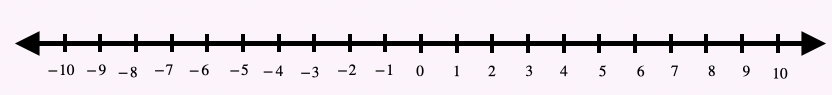## Introduction

• Integers are a set of whole numbers (0, 1, 2, 3, ....) and their opposites. For example, 1, -1,  2, -2, 3, -3, and so on.
• 0 is an integer. It is also called the origin. 0 does not carry any sign. When any integer is added to 0, the answer is the same number. e.g.
• The integer with a positive sign is greater than 0 and is to the right of 0 on the number line.
• The integer with a negative sign is less than 0 and is to the left of 0 on the number line.• In a vertical number line, numbers above 0 are positive, and numbers below zero are negative.

• Two integers are opposite if they have different signs and exactly the same distance from 0, such as
• The opposite of an opposite is a positive, for e.g. .
• The additive inverse is when a number and its opposite are added resulting in "0". E.g. .

• Rule 1: When adding two integers with the same signs, add the numbers and keep the same sign.
• Rule 2: When adding two integers with different signs, subtract the numbers and keep the sign of the greater number.

## Subtracting Integers

• Rule: When subtracting two integers:

First, change the subtraction sign to the addition.

Next, change the sign of the number that is being subtracted.

• Follow the order of operations when working with more than two integers.

## Solved Examples

Example 1: What is the opposite of -9?

Solution: The opposite of -9 is 9, as it has exactly the same distance from 0.

Example 2: What is the value of -(-24)?

Solution: The opposite of opposite is positive.

-(-24) = 24

Solution:  Use the rule for adding integers with the same sign.

Solution: Use the rule for the addition of integers with different signs.

Example 5: Subtract:

Solution: Use the rule for the subtraction of the integers.

Example 6: Subtract:

Solution: Use the rule for the subtraction of the integers.

Example 7: Compare:

Solution: , as -3 is at the right of -10 on a number line.

## Cheat Sheet

• A number without any sign is considered positive (except 0, as 0 is neither positive nor negative).